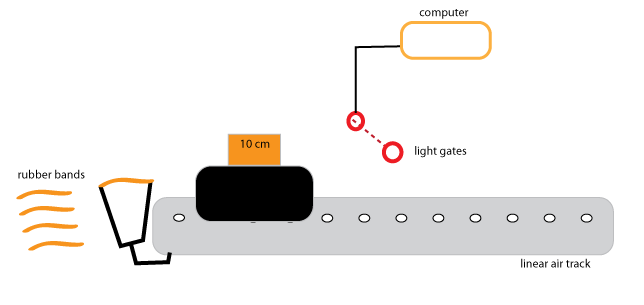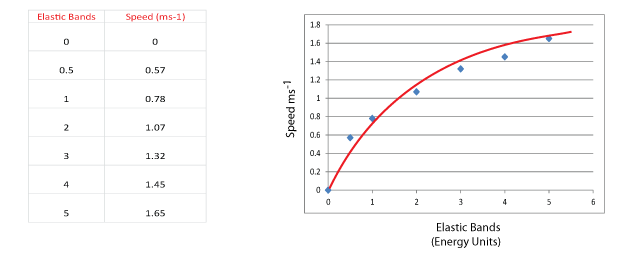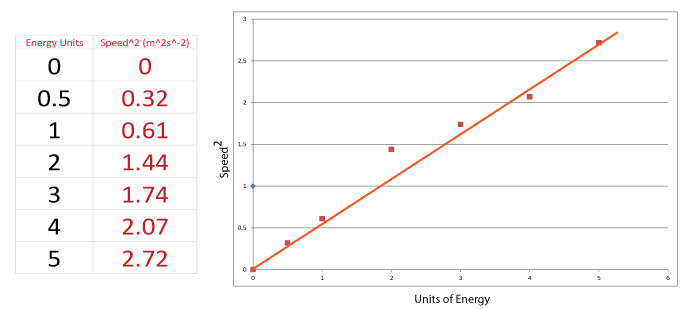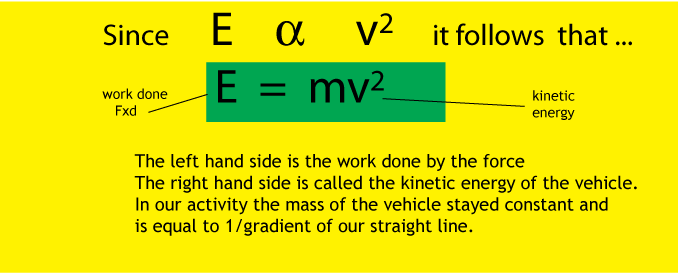# Unit 2 Electricity and Energy

Learning Outcomes

Homeworks

Click for Homework on Electrical Circuits

Click for Revision for test

Power Homework

Click for Electricity and Energy Formal Homework 1

Click for Conservation of Energy Formal Homework

Click for Gases Homework

Click for Gases Homework 2

## The Conservation of Energy

You will have noticed by this that the study of Physics is the study of energy. Energy comes in many forms and are interchangeable. This means that energy can be changed from one form to another. For example a solar cell changes light energy to electrical energy. We also know that during this process that 100% of the light energy absorbed by the solar cell will be changed to electrical energy and other forms, for example heat. This is known as the Law of Conservation of Energy. Our first investigation is to test this law using the work done by elastic bands on a vehicle and comparing this with the kinetic energy of the vehicle. We used the apparatus shown below.We pushed the vehicle against the rubber bands. The rubber bands pushed the vehicle to a top speed which was calculated by a computer connected to a pair of light gates.
This was our results.As you can see we get a curve. However before we can make proper predictions we need to get a straight line through the origin, we can then use what we know about straight lines from our maths classes and find a formula.
If we square all the speeds and plot those values with the energy units then we get a straight line graph through the origin. This means the square of a body's speed is directly proportional to the energy it has been supplied with.Task It is now up to us to verify that this formula is correct. The energy supplied to the vehicle was supplied by elastic bands. When we used 5 bands it exerted a maximum force of 15 N and it pushed the 0.24 kg vehicle through a distance of 0.06 m and it reached a speed of 1.65 ms-1. Find the average force the bands exerted on the vehicle over this distance then find the work done (Average Force x Distance) on the vehicle. After that check it is the same as the kinetic energy of the vehicle. If the kinetic energy is slightly less than the work done then this can be explained away using energy losses. If the kinetic energy is greater than the work done by the bands then we have a violation of the Conservation of Energy and it is back to the drawing board.Click for homework suggestion

Click for NAT 4 homework suggestion

Click for Information on Car Safety task

Click for Candidates Guide for Car Safety task